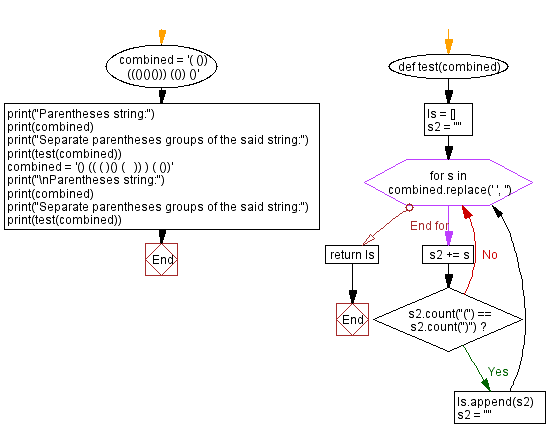﻿ Python: Separate parentheses groups - w3resource

# Python: Separate parentheses groups

## Python Programming Puzzles: Exercise-94 with Solution

Given a string consisting of whitespace and groups of matched parentheses, write a Python program to split it into groups of perfectly matched parentheses without any whitespace.

```Input:
( ()) ((()()())) (()) ()
Output:
['(())', '((()()()))', '(())', '()']

Input:
() (( ( )() (   )) ) ( ())
Output:
['()', '((()()()))', '(())']
```

Sample Solution:

Python Code:

``````#License: https://bit.ly/3oLErEI

def test(combined):
ls = []
s2 = ""
for s in combined.replace(' ', ''):
s2 += s
if s2.count("(") == s2.count(")"):
ls.append(s2)
s2 = ""
return ls
combined = '( ()) ((()()())) (()) ()'
print("Parentheses string:")
print(combined)
print("Separate parentheses groups of the said string:")
print(test(combined))
combined = '() (( ( )() (   )) ) ( ())'
print("\nParentheses string:")
print(combined)
print("Separate parentheses groups of the said string:")
print(test(combined))
``````

Sample Output:

```Parentheses string:
( ()) ((()()())) (()) ()
Separate parentheses groups of the said string:
['(())', '((()()()))', '(())', '()']

Parentheses string:
() (( ( )() (   )) ) ( ())
Separate parentheses groups of the said string:
['()', '((()()()))', '(())']
```

Flowchart:Python Code Editor :

Have another way to solve this solution? Contribute your code (and comments) through Disqus.

What is the difficulty level of this exercise?

Test your Programming skills with w3resource's quiz.

﻿